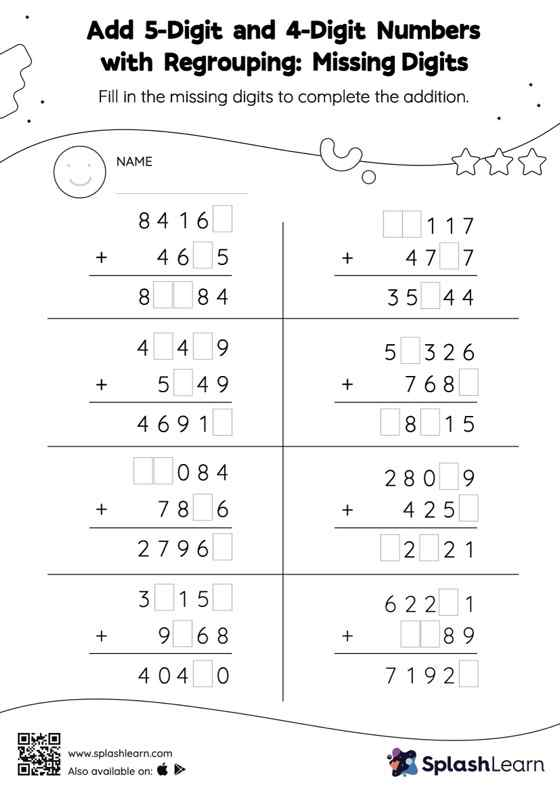# Add 5-Digit and 4-Digit Numbers with Regrouping: Missing Digits Worksheet

Home > Add 5-Digit and 4-Digit Numbers with Regrouping: Missing DigitsChallenge your young math wizards to solve this add 5-digit and 4-digit numbers with regrouping worksheet. Students use the count on strategy or the relationship between addition and subtraction to find the missing number in this worksheet. They also need to regroup numbers to get to the answer in add 5-digit and 4-digit numbers with regrouping worksheet. This worksheet is about practicing the column method in which numbers are written one on top of another. In this method, students use their place value understanding to solve the problems.